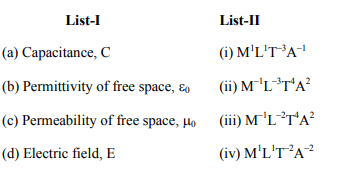Deepak Scored 45->99%ile with Bounce Back Crack Course. You can do it too!

# Match List I with List II

Question:

Match List I with List IIChoose the correct answer from the options given below

1. (a) $\rightarrow$ (iii), (b) $\rightarrow$ (ii), (c) $\rightarrow$ (iv), (d) $\rightarrow$ (i)

2. (a) $\rightarrow$ (iii), (b) $\rightarrow$ (iv), (c) $\rightarrow$ (ii), (d) $\rightarrow$ (i)

3. (a) $\rightarrow$ (iv), (b) $\rightarrow$ (ii), (c) $\rightarrow$ (iii), (d) $\rightarrow$ (i)

4. (a) $\rightarrow$ (iv), (b) $\rightarrow$ (iii), (c) $\rightarrow($ ii $)$, (d) $\rightarrow$ (i)

Correct Option: 1

Solution:

q = CV

$[\mathrm{C}]=\left[\frac{\mathrm{q}}{\mathrm{V}}\right]=\frac{(\mathrm{A} \times \mathrm{T})^{2}}{\mathrm{ML}^{2} \mathrm{~T}^{-2}}$

$=\mathrm{M}^{-1} \mathrm{~L}^{-2} \mathrm{~T}^{4} \mathrm{~A}^{2}$

$[\mathrm{E}]=\left[\frac{\mathrm{F}}{\mathrm{q}}\right]=\frac{\mathrm{MLT}^{-2}}{\mathrm{AT}}$

$=\mathrm{MLT}^{-3} \mathrm{~A}^{-1}$

$\mathrm{F}=\frac{\mathrm{q}_{1} \mathrm{q}_{2}}{4 \pi \epsilon_{\mathrm{o}} \mathrm{r}^{2}}$

$\left[\epsilon_{o}\right]=M^{-1} L^{-3} T^{4} A^{2}$

Speed of light $\mathrm{c}=\frac{1}{\sqrt{\mu_{\mathrm{o}} \epsilon_{0}}}$

$\mu_{0}=\frac{1}{\epsilon_{0} \mathrm{c}^{2}}$

$\left[\mu_{0}\right]=\frac{1}{\left[\mathrm{M}^{-1} \mathrm{~L}^{-3} \mathrm{~T}^{4} \mathrm{~A}^{2}\right]\left[\mathrm{LT}^{-1}\right]^{2}}$

$=\left[\mathrm{M}^{1} \mathrm{~L}^{1} \mathrm{~T}^{-2} \mathrm{~A}^{-2}\right]$